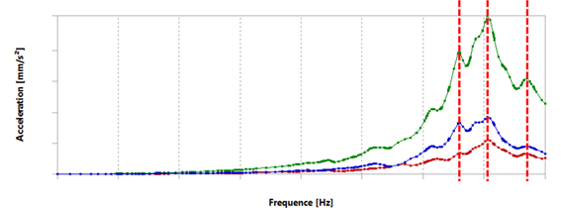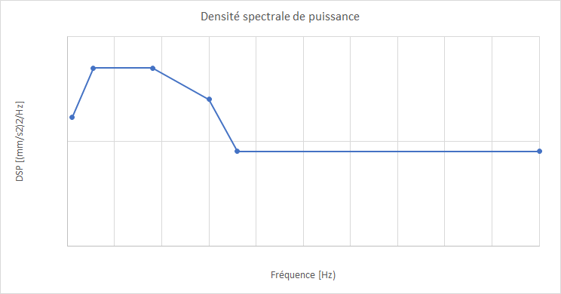Phimeca carried out vibratory calculations on an inverter undergoing random changes (PSD). The purpose of this study is to estimate the level of damage on the whole inverter after severe tests, representative of a life cycle of the product.

During a random vibration test, the most important risks of fracture are due to the fatigue. One of the methods that allows an estimation of the damages is based on the Steinberg’s three-band method that hypothesizes a Gaussian normal distribution of the stress. This theory states that:

• 3 % of the time, the constraints are inferior to the constraints RMS response
• 1 % of the time, the constraints are superior to the constraints RMS response and inferior to the double of the constraints RMS response
• 33 % of the time, the constraints are superior to the double of the constraints RMS response and inferior to the triple of the constraints RMS response

Using the Wöhler curve of the material and the three calculated constraints, the number of cycles that the structure can withstand while undergoing each of these three constraints is calculated.

Considering the observed test duration, the ratio of cumulative fatigue damage is calculated on the structure. If this ratio is superior or equal to 1, the structure will not hold during the test.

Main operations

• Finite-element modelling of the inverter
• Modal basis calculation and DSP vibratory calculations
• Mechanical stress calculations on the different parts of the inverter
• Estimation of the level of damage on the different parts of the inverterFrequency response curve (calculated acceleration)PSD injected

Tool: Ansys